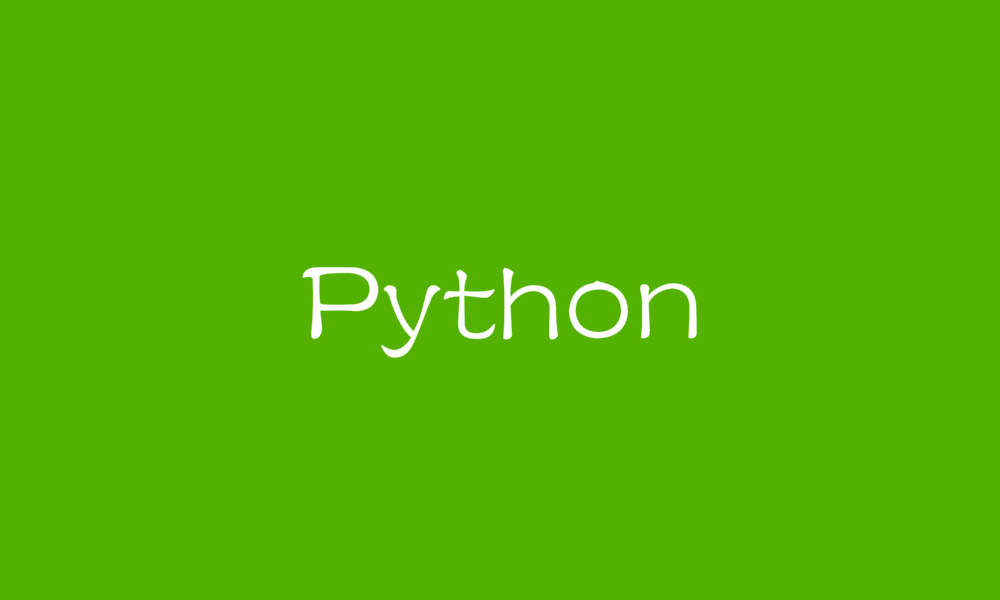# Python 多进程之间共享变量AlwaysBeta﻿

Python 多线程之间共享变量很简单，直接定义全局 global 变量即可。而多进程之间是相互独立的执行单元，这种方法就不可行了。

﻿

﻿

### Process 多进程

﻿

﻿

import multiprocessingdef func(num):    # 共享数值型变量    # num.value = 2    # 共享数组型变量    num = 9999if __name__ == '__main__':    # 共享数值型变量    # num = multiprocessing.Value('d', 1)    # print(num.value)    # 共享数组型变量    num = multiprocessing.Array('i', [1, 2, 3, 4, 5])    print(num[:])    p = multiprocessing.Process(target=func, args=(num,))    p.start()    p.join()    # 共享数值型变量    # print(num.value)    # 共享数组型变量    print(num[:])

﻿

### Pool 进程池

﻿

﻿

from multiprocessing import Pool, Managerdef func(my_list, my_dict):    my_list.append(10)    my_list.append(11)    my_dict['a'] = 1    my_dict['b'] = 2if __name__ == '__main__':    manager = Manager()    my_list = manager.list()    my_dict = manager.dict()    pool = Pool(processes=2)    for i in range(0, 2):        pool.apply_async(func, (my_list, my_dict))    pool.close()    pool.join()    print(my_list)    print(my_dict)

﻿

﻿

def func(my_list, my_dict):    my_list = [10, 11]    my_dict['a'] = 1    my_dict['b'] = 2

﻿

﻿

﻿

https://blog.csdn.net/houyanhua1/article/details/78244288

﻿## 评论